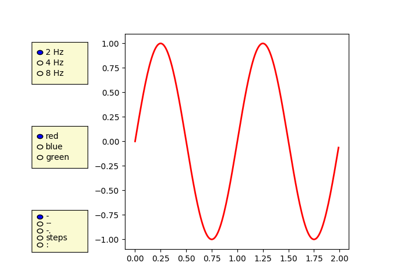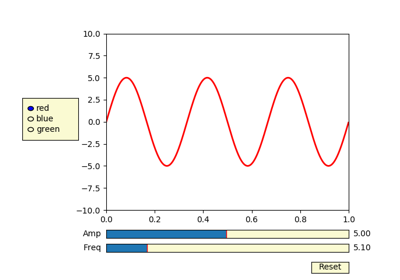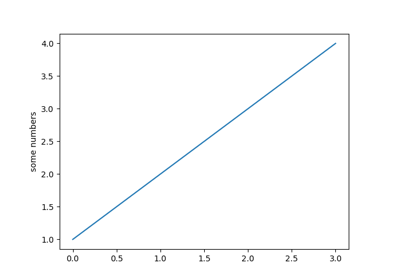### Related Topics

`matplotlib.pyplot.``subplots_adjust`(*args, **kwargs)[source]

Tune the subplot layout.

call signature:

```subplots_adjust(left=None, bottom=None, right=None, top=None,
wspace=None, hspace=None)
```

The parameter meanings (and suggested defaults) are:

```left  = 0.125  # the left side of the subplots of the figure
right = 0.9    # the right side of the subplots of the figure
bottom = 0.1   # the bottom of the subplots of the figure
top = 0.9      # the top of the subplots of the figure
wspace = 0.2   # the amount of width reserved for space between subplots,
# expressed as a fraction of the average axis width
hspace = 0.2   # the amount of height reserved for space between subplots,
# expressed as a fraction of the average axis height
```

The actual defaults are controlled by the rc file

## Examples using `matplotlib.pyplot.subplots_adjust`¶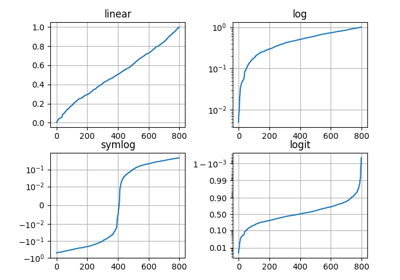Pyplot Scales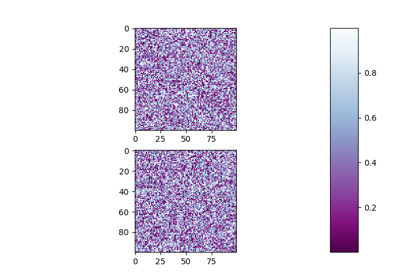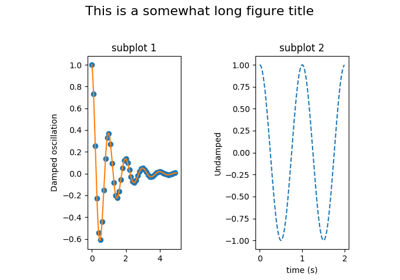Figure Title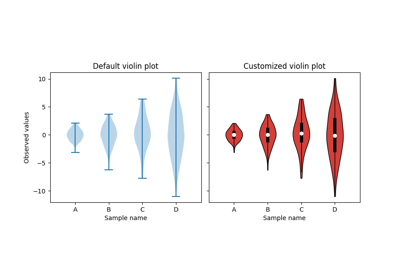Violin plot customization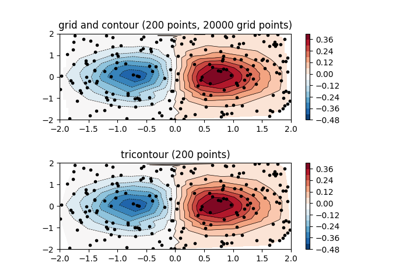Contour plot of irregularly spaced data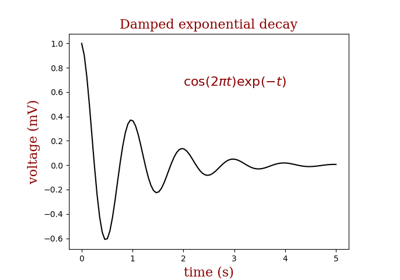Controlling style of text and labels using a dictionary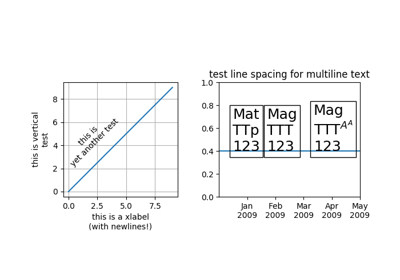Multiline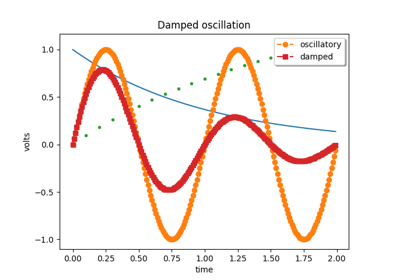Legend Demo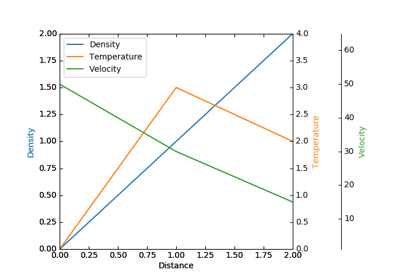Demo Parasite Axes2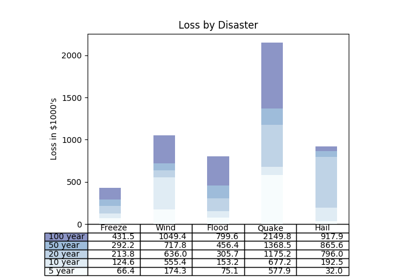Table Demo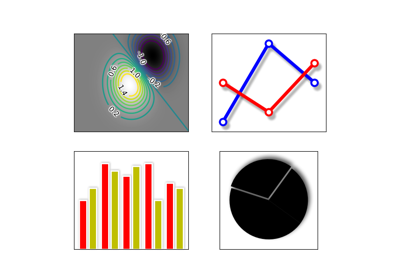Demo Agg Filter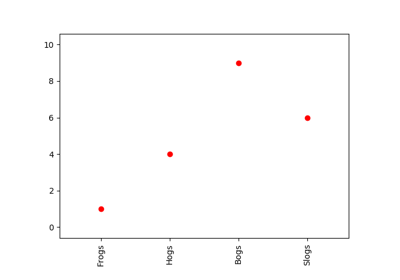Rotating custom tick labels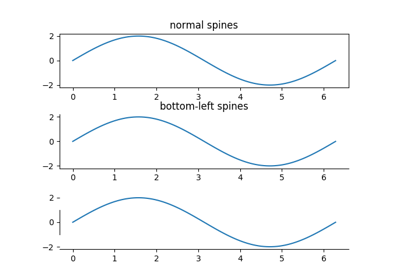Spines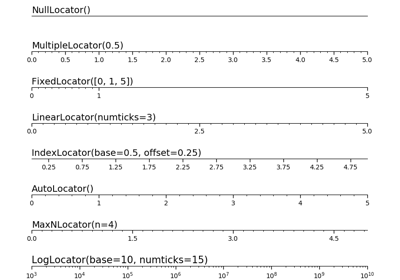Tick locators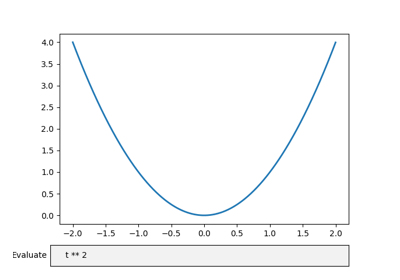Textbox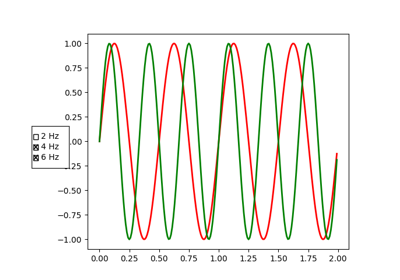Check Buttons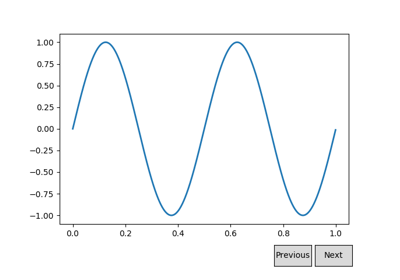Buttons